# RS Aggarwal Solutions for Class 9 Chapter 12: Circles Exercise 12B

## RS Aggarwal Solutions for Class 9 Maths Exercise 12B PDF

Appearing for the board exams is a nervous task for all the students. Scoring good marks in a subject like Mathematics is difficult until the students understand the concepts. RS Aggarwal Solutions are prepared with the aim of helping students to be clear with the topics which are covered in each chapter. The solutions are explained in an interactive manner to make it interesting for the students while studying. Solving the RS Aggarwal textbook has become easier with the help of solutions which are prepared by subject matter experts. RS Aggarwal Solutions for Class 9 Maths Chapter 12 Circles Exercise 12B are provided here.

## RS Aggarwal Solutions for Class 9 Chapter 12: Circles Exercise 12B Download PDF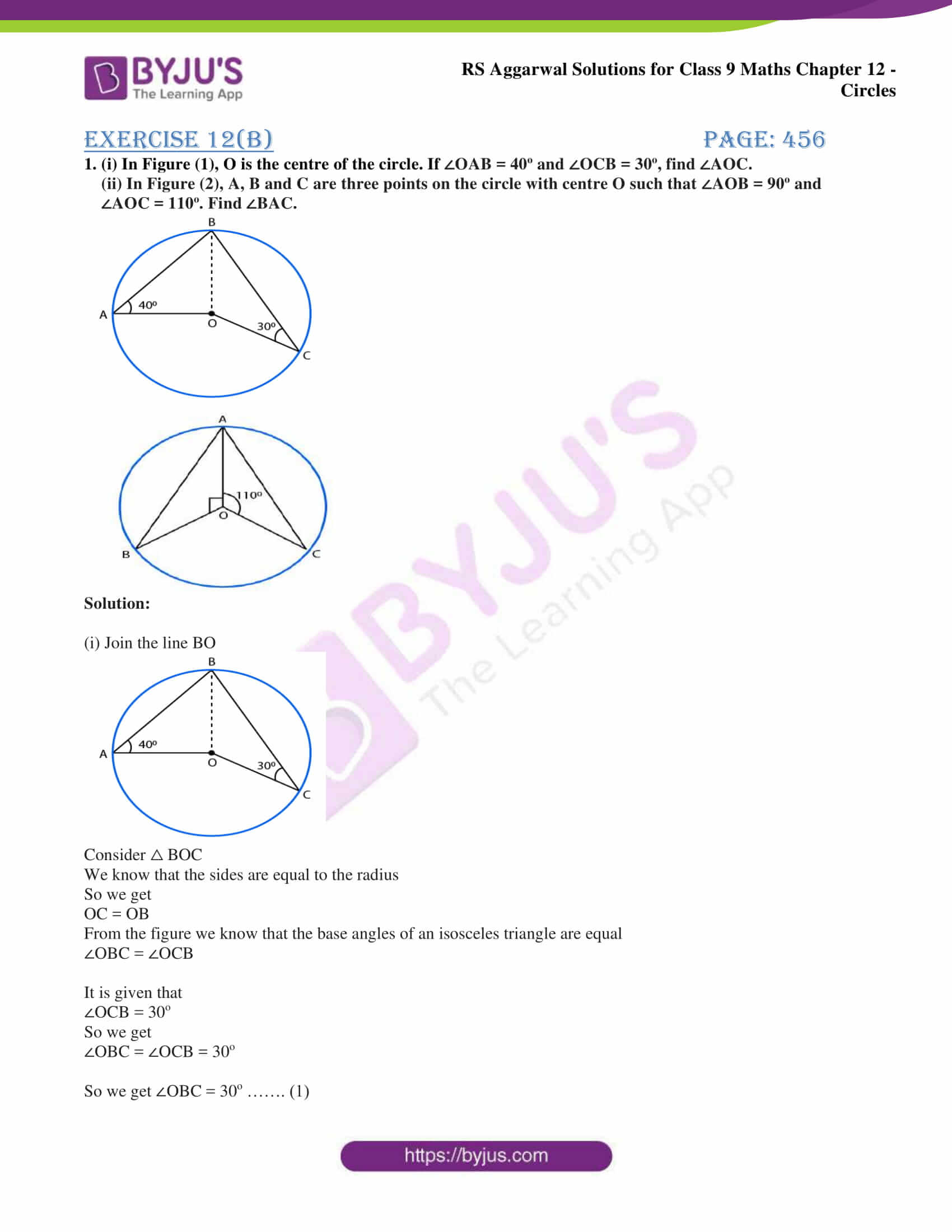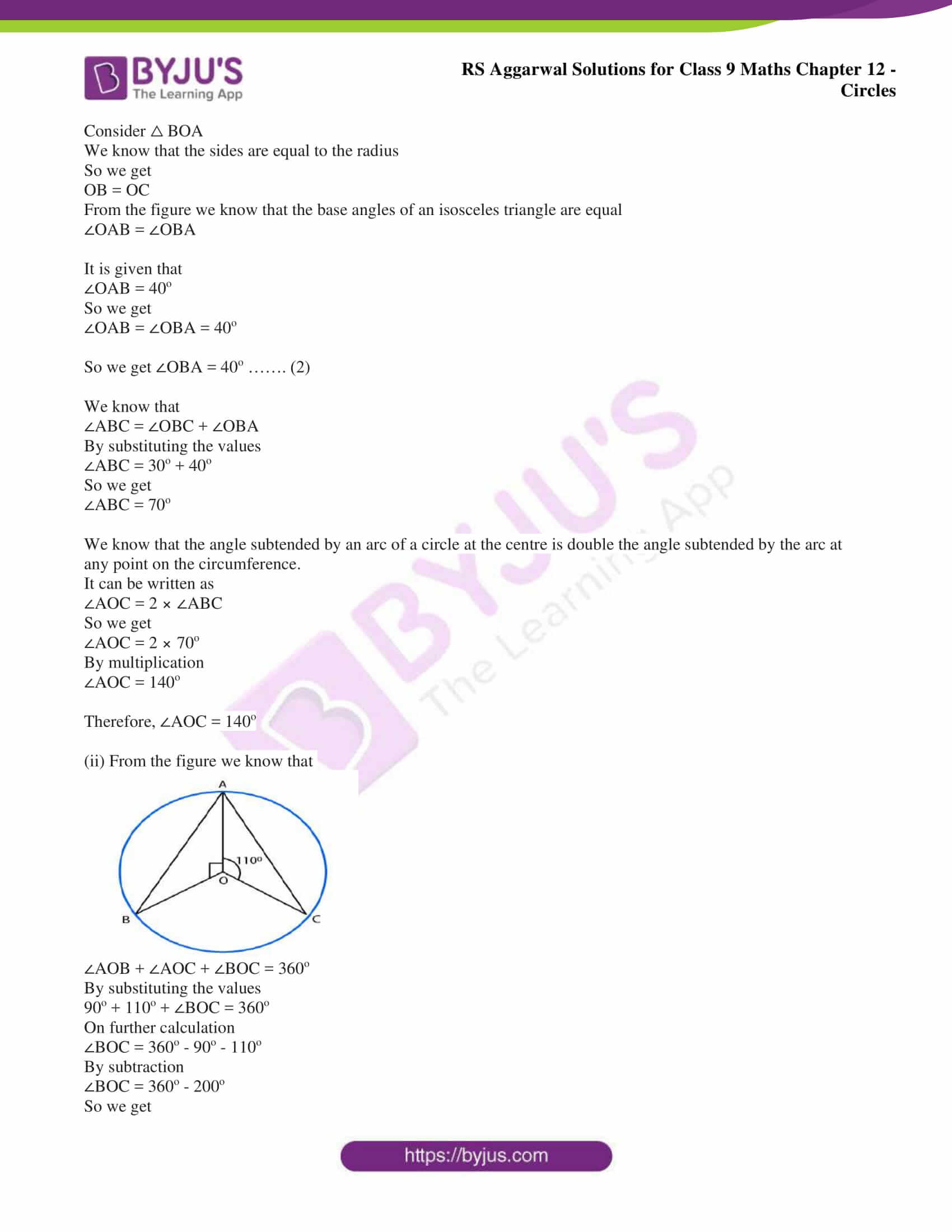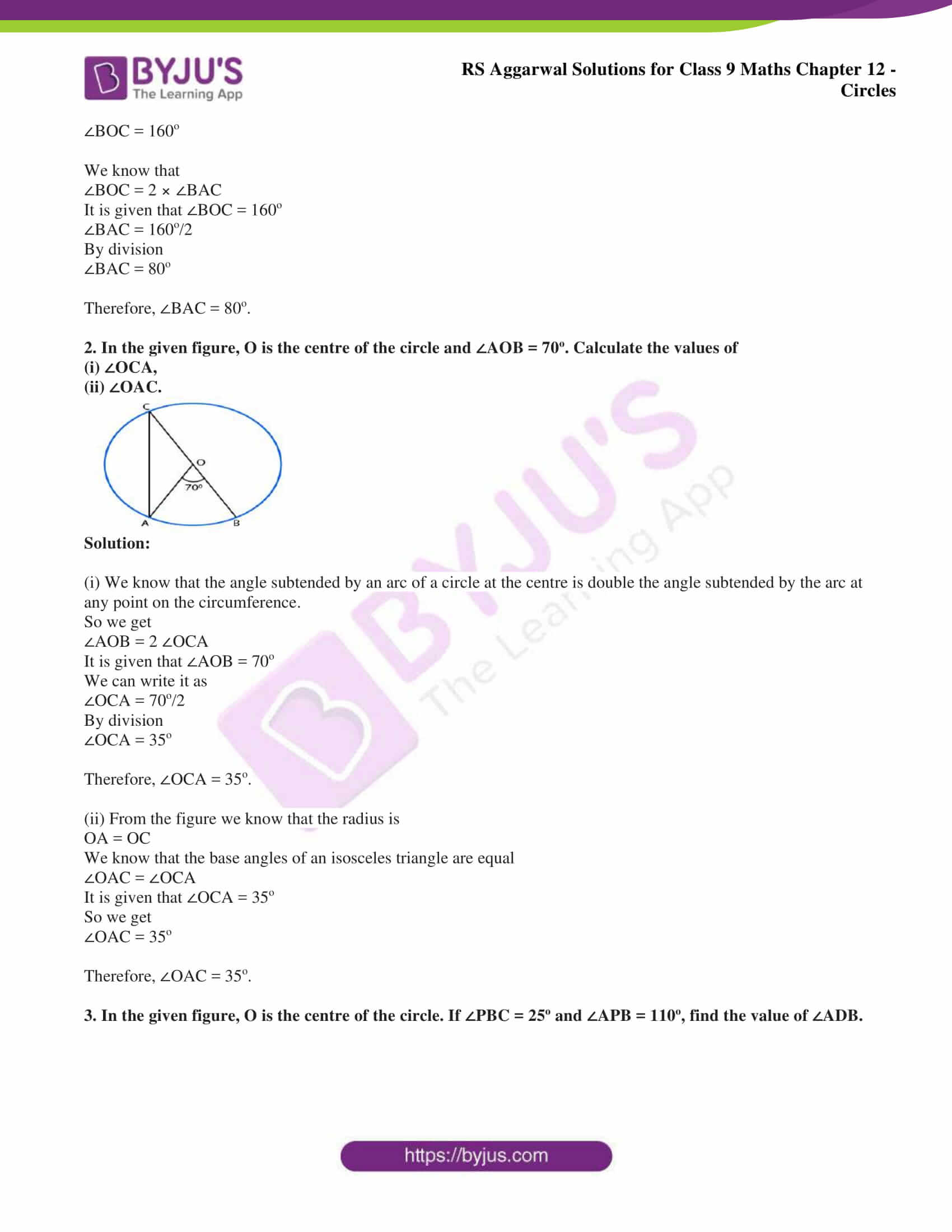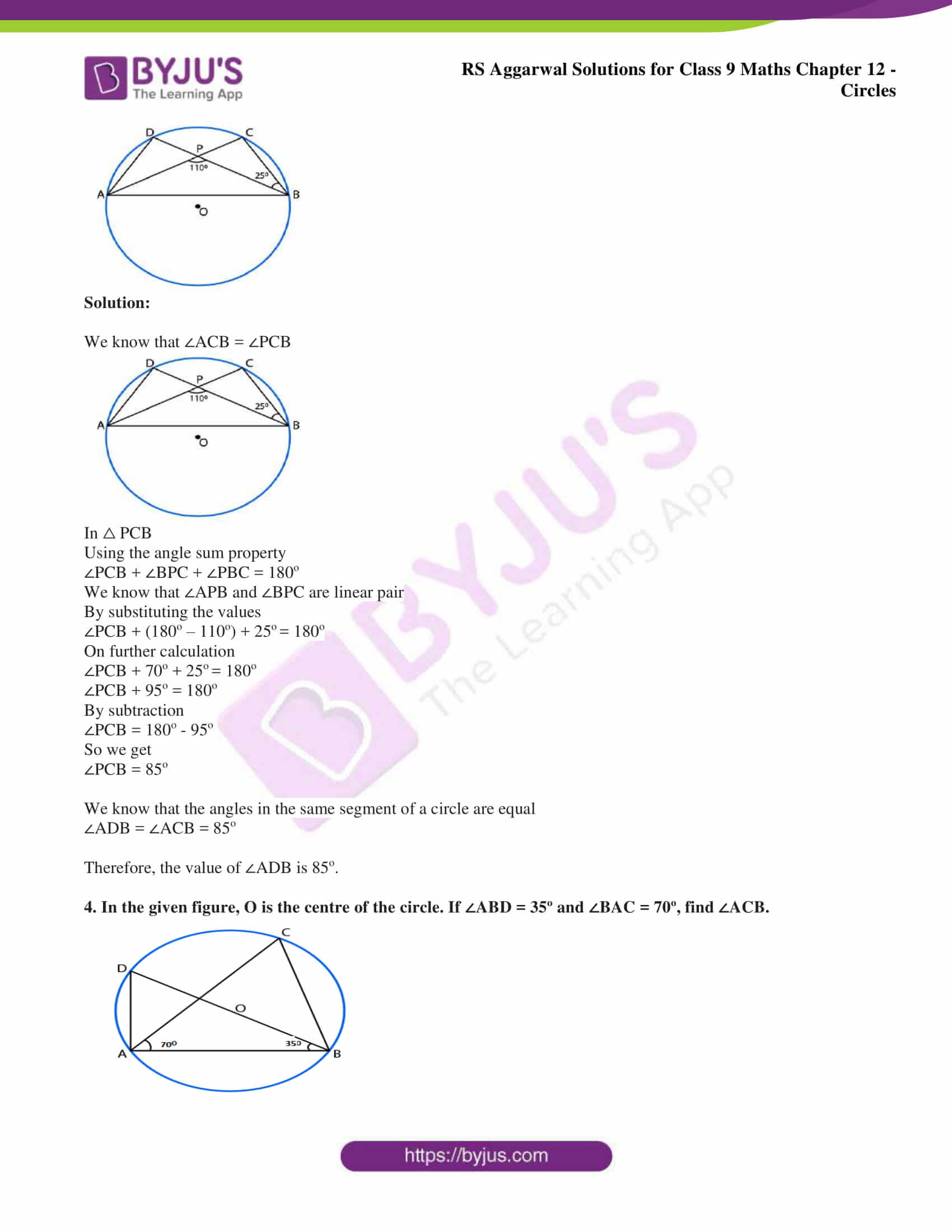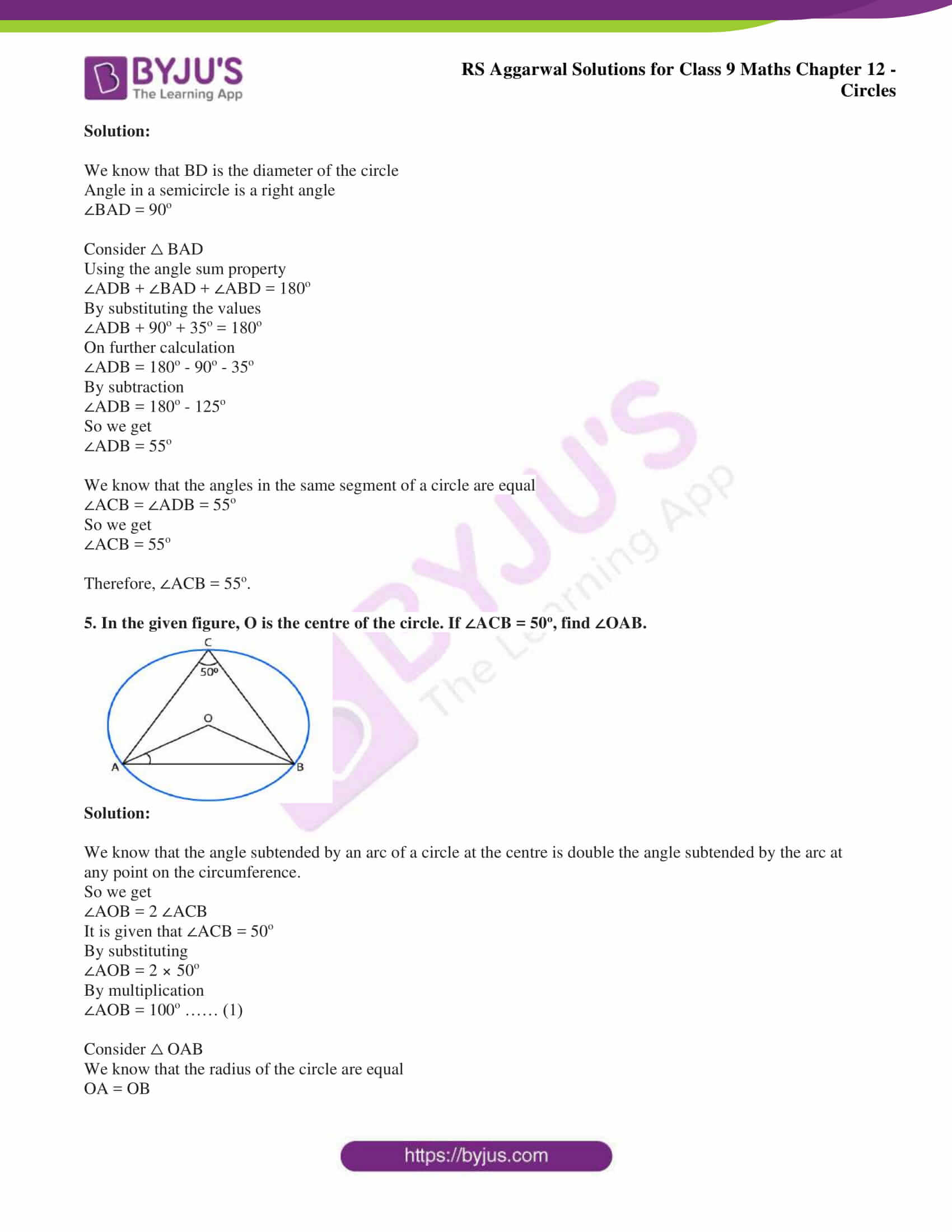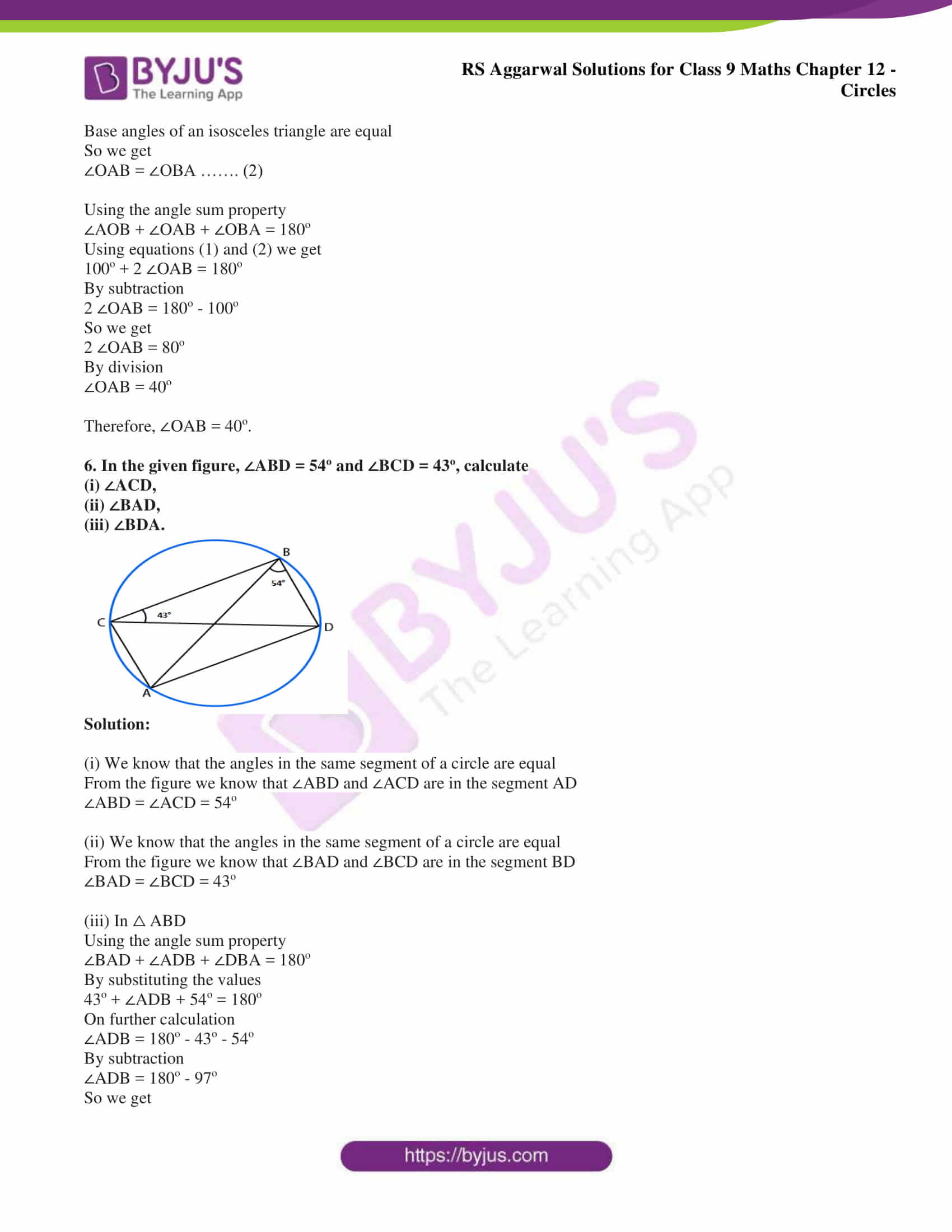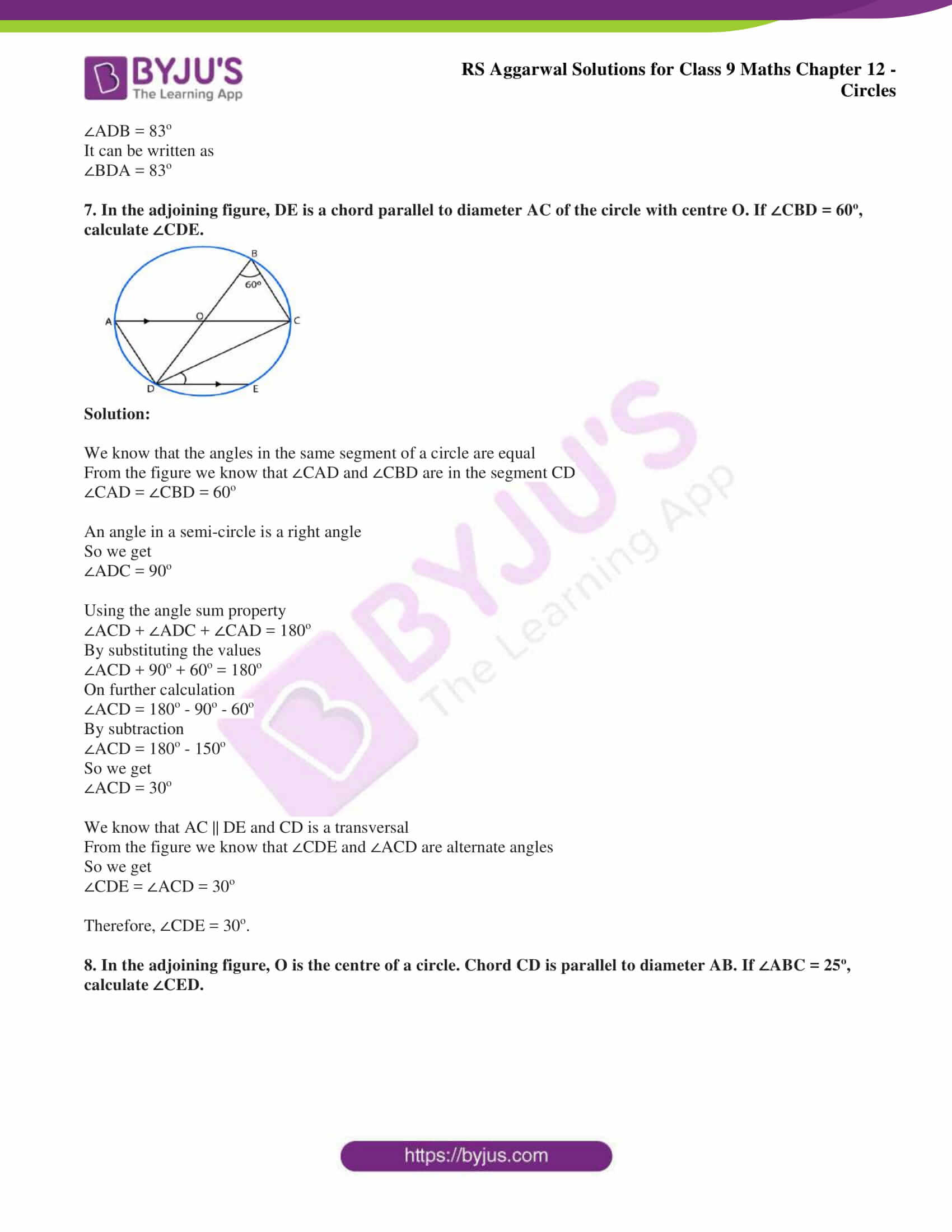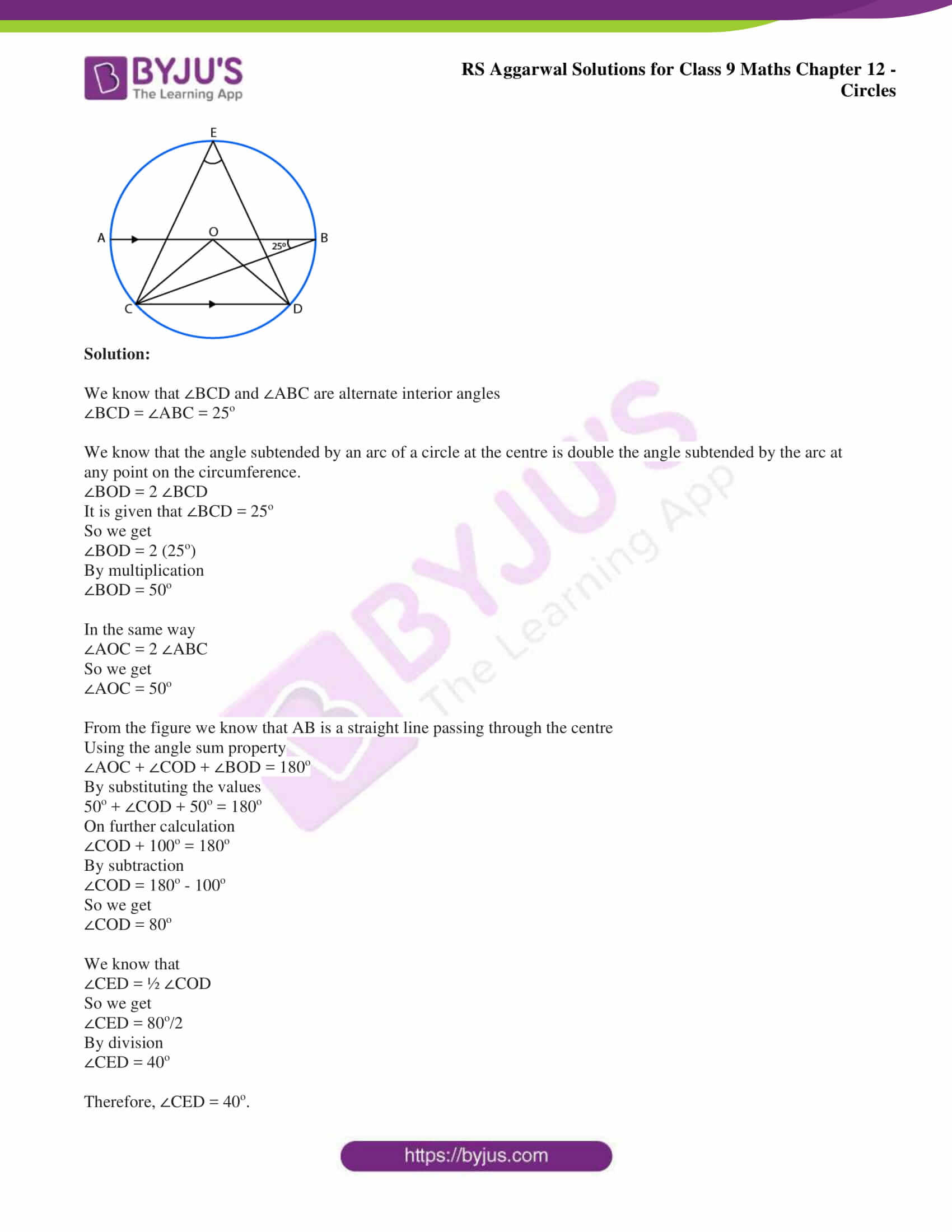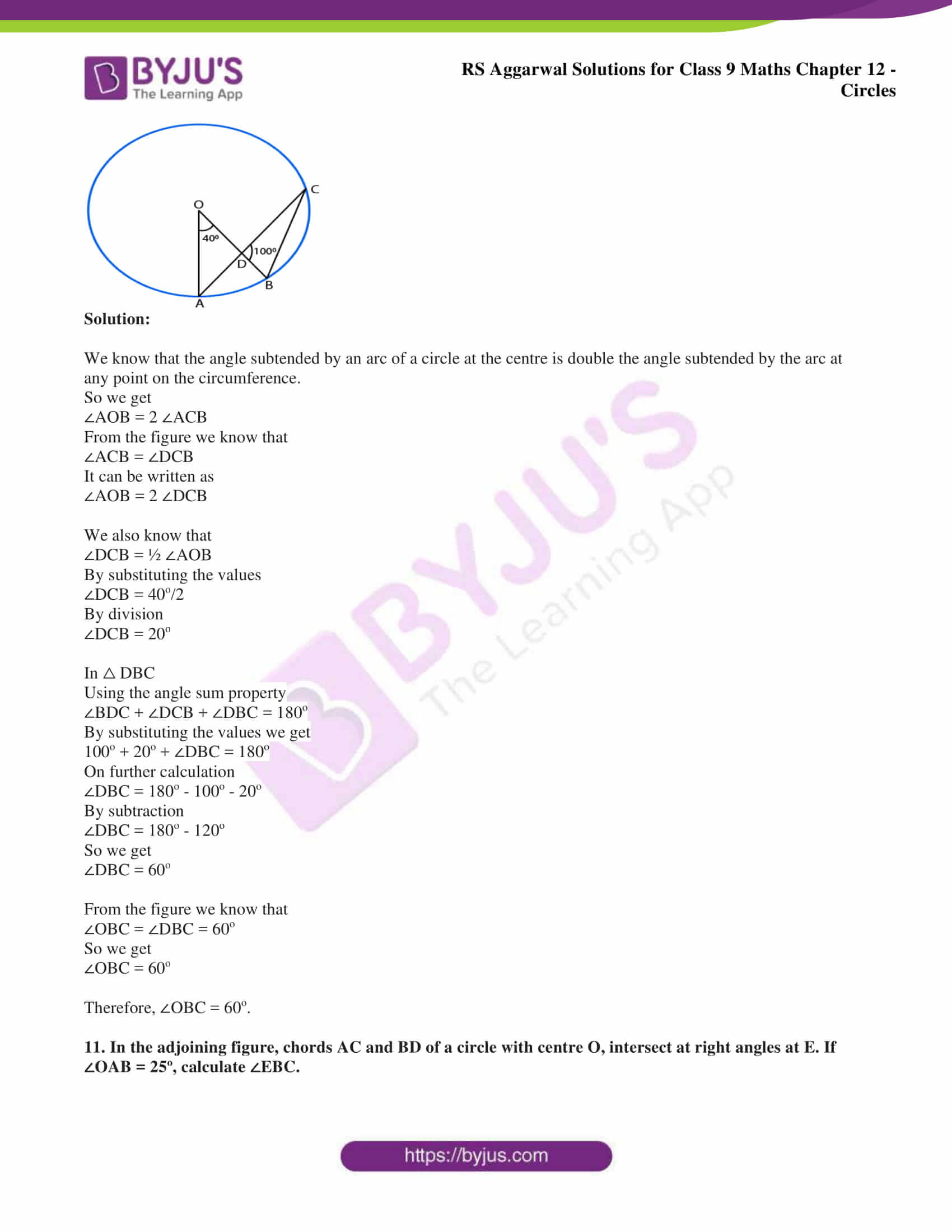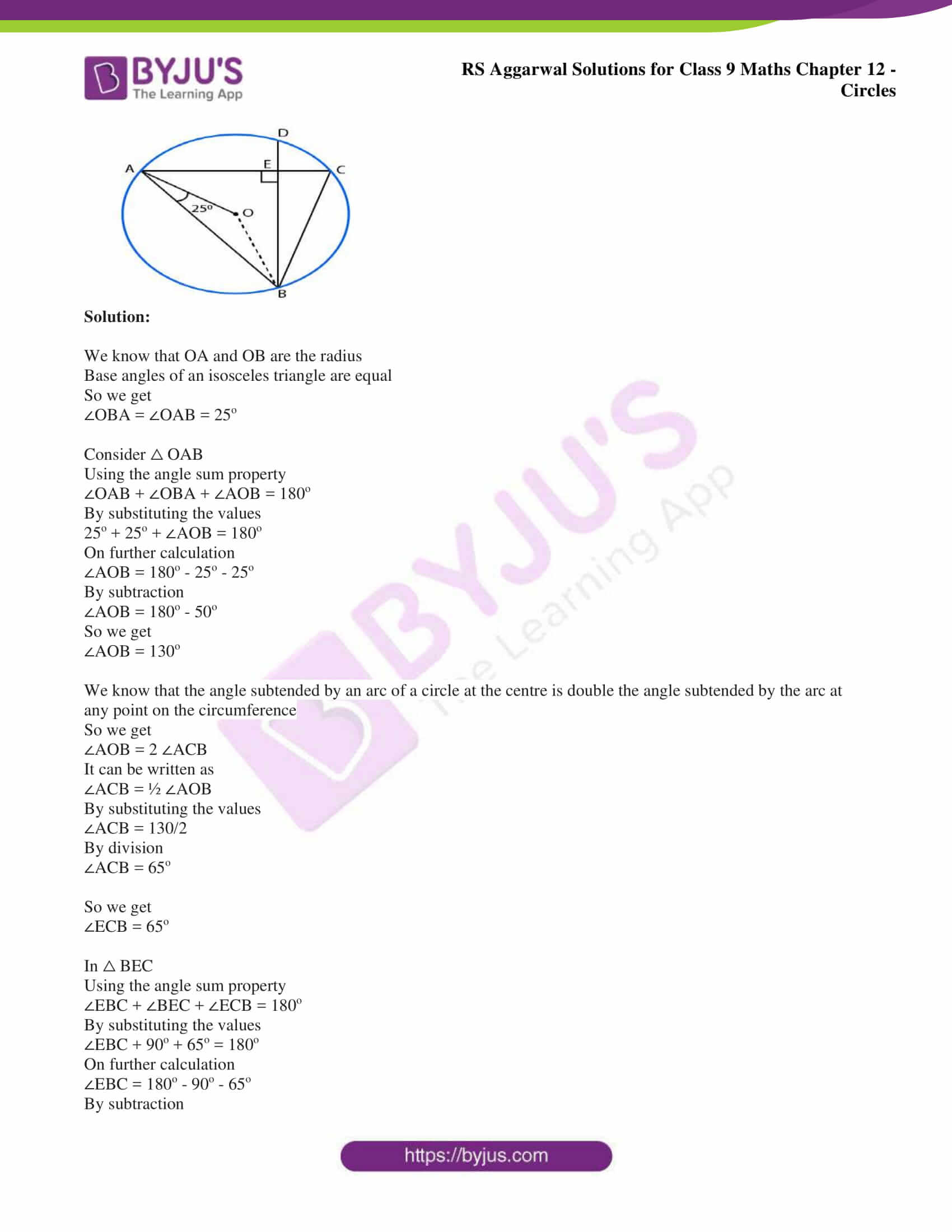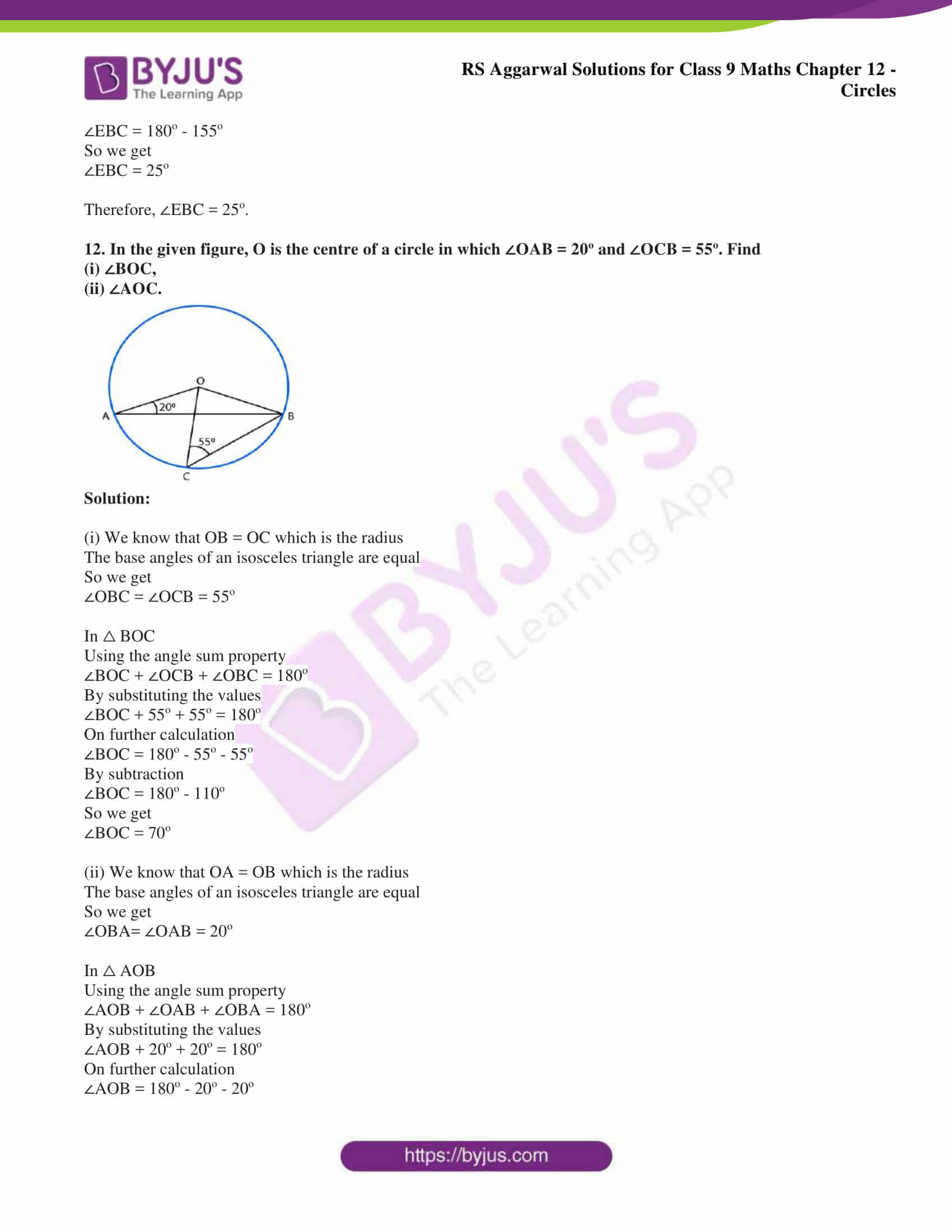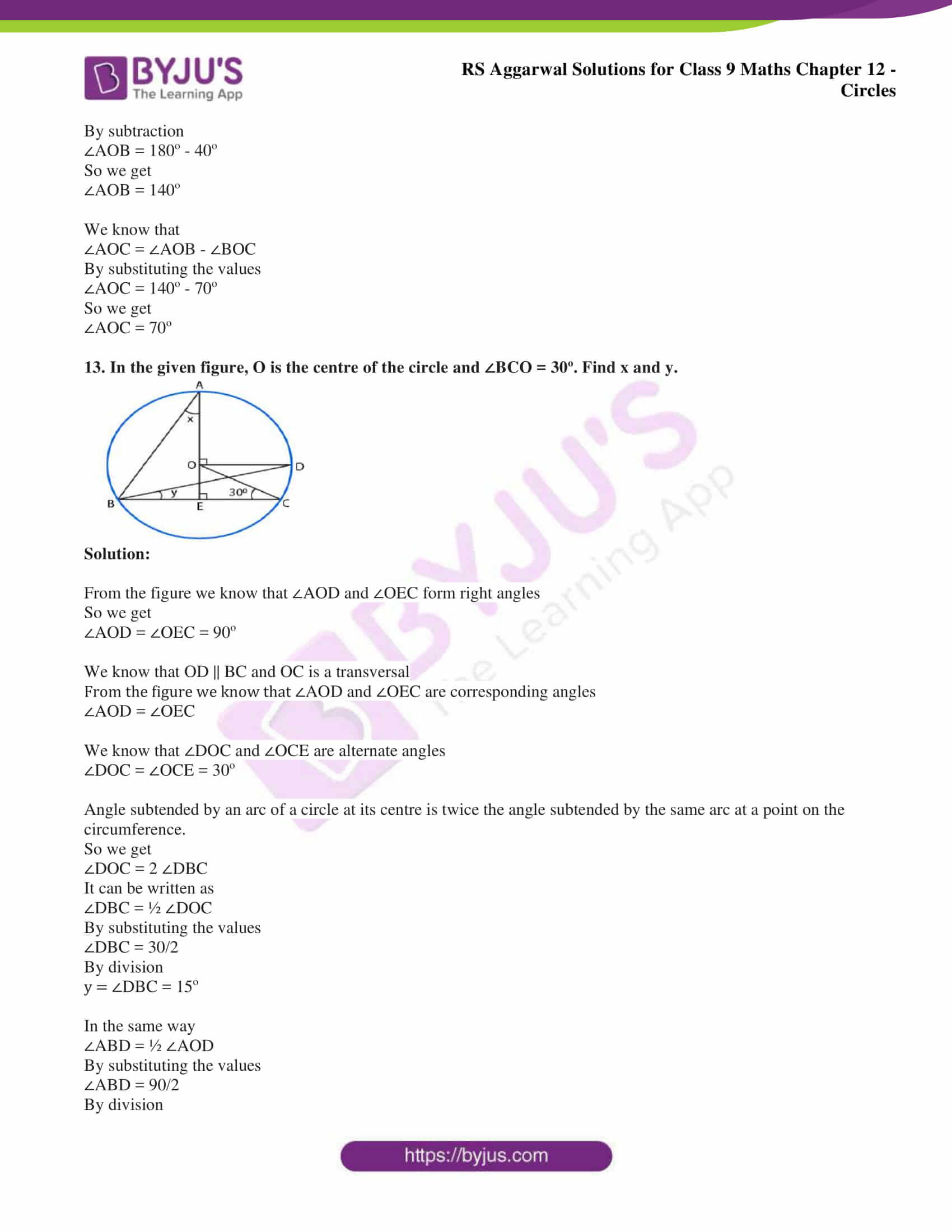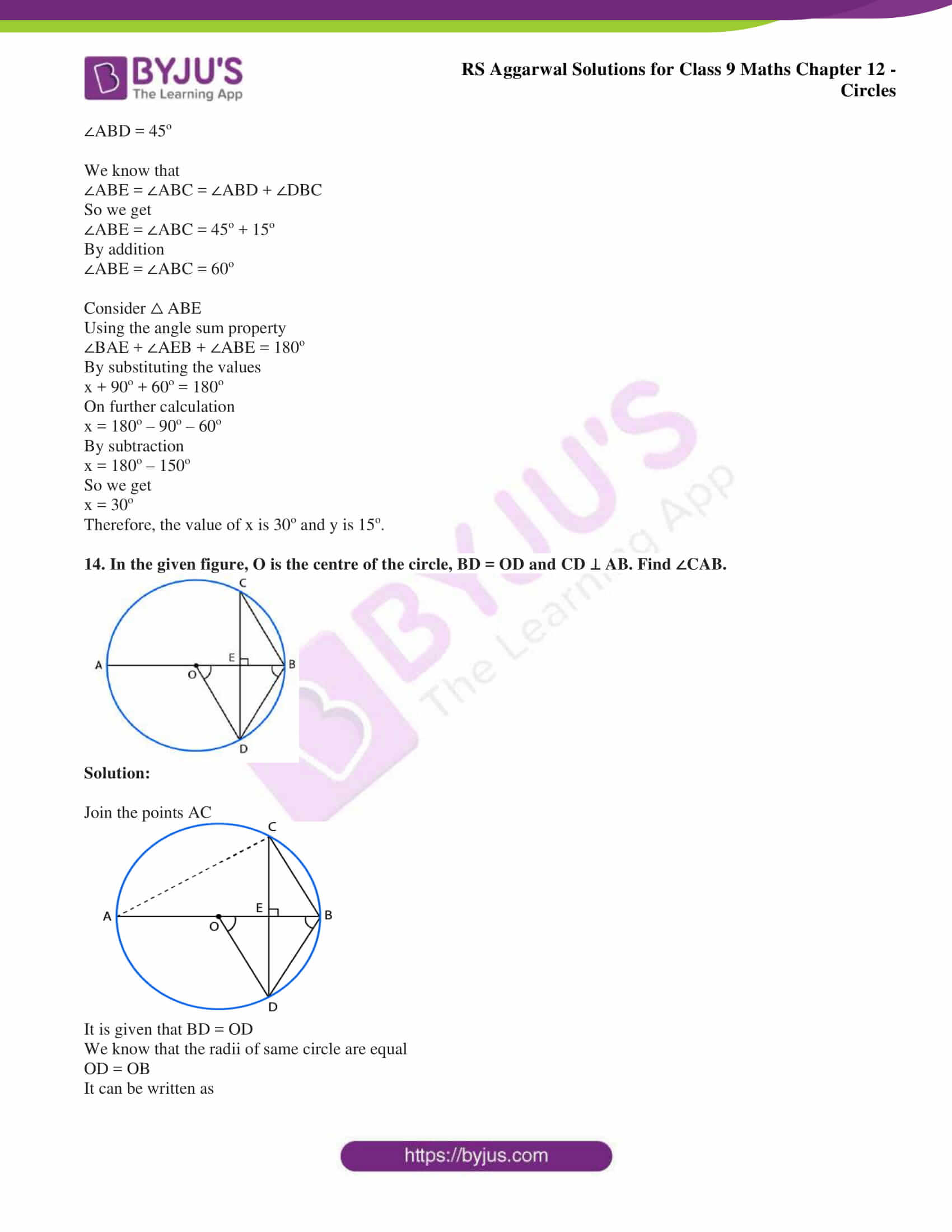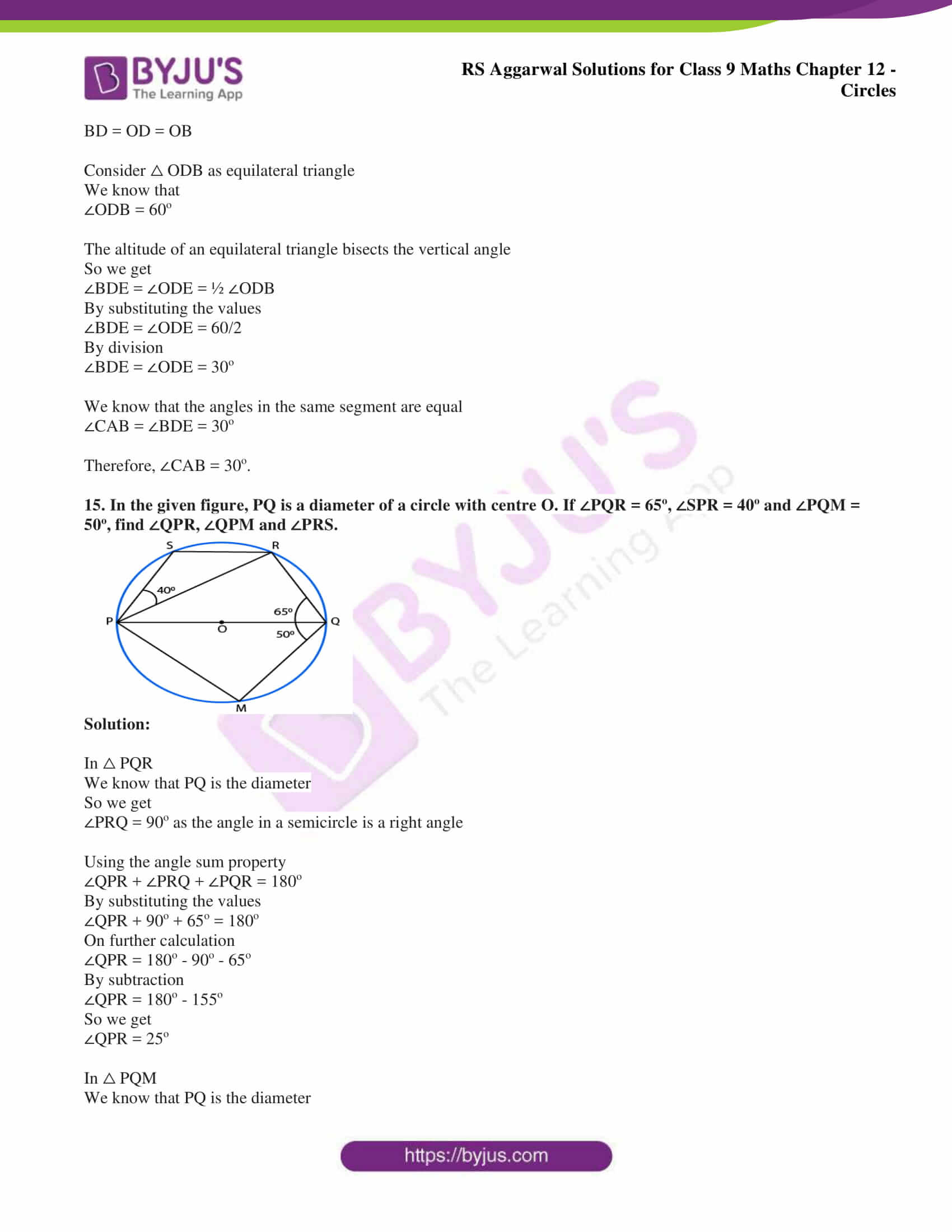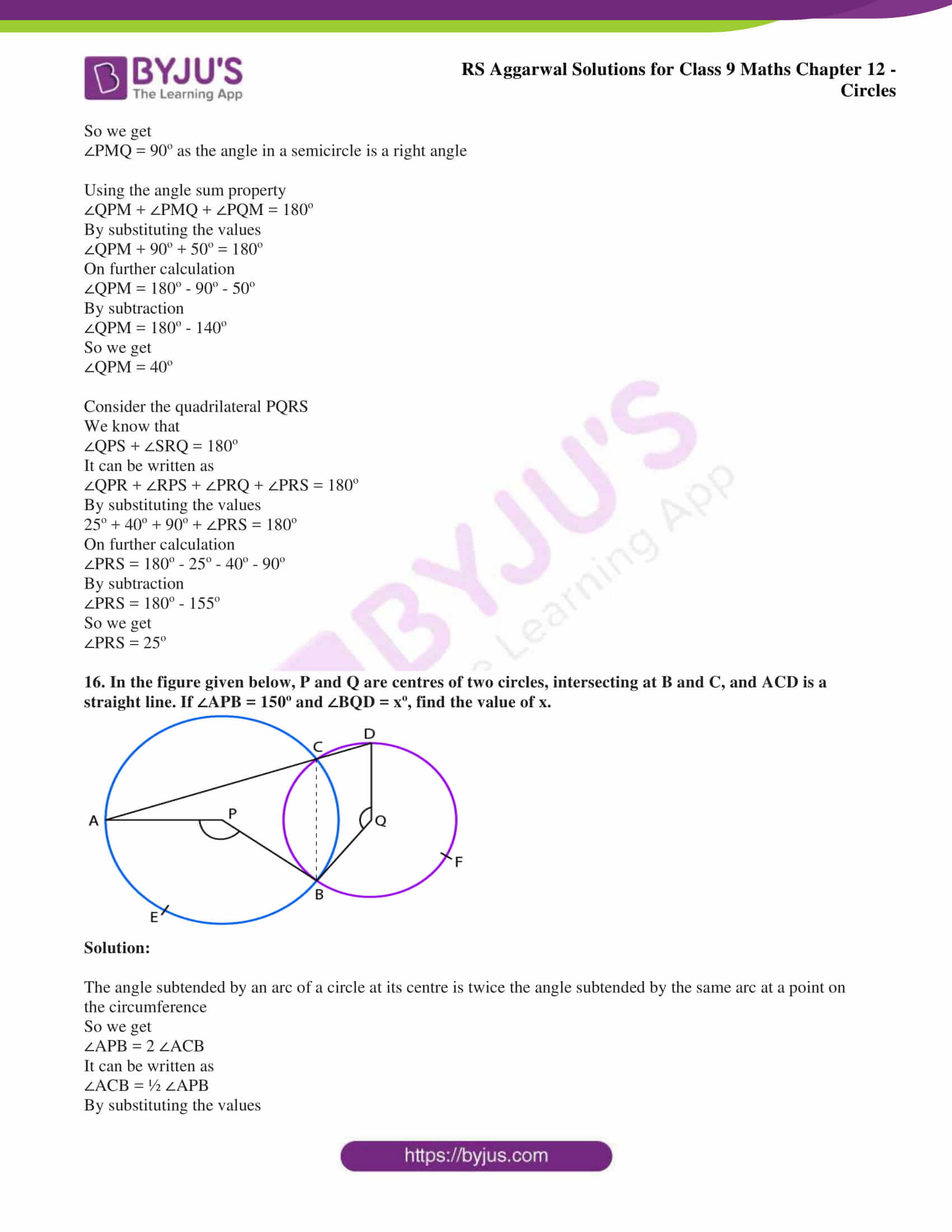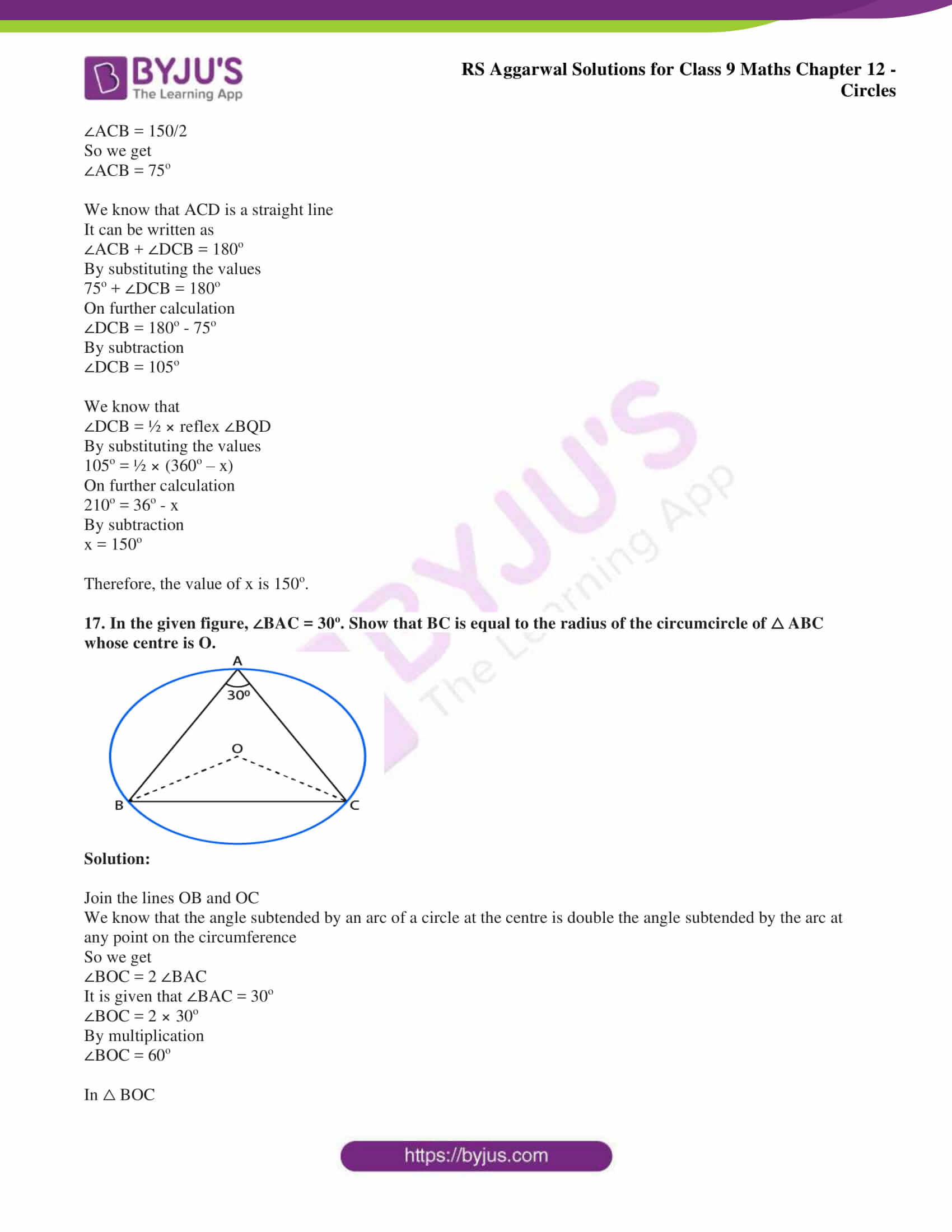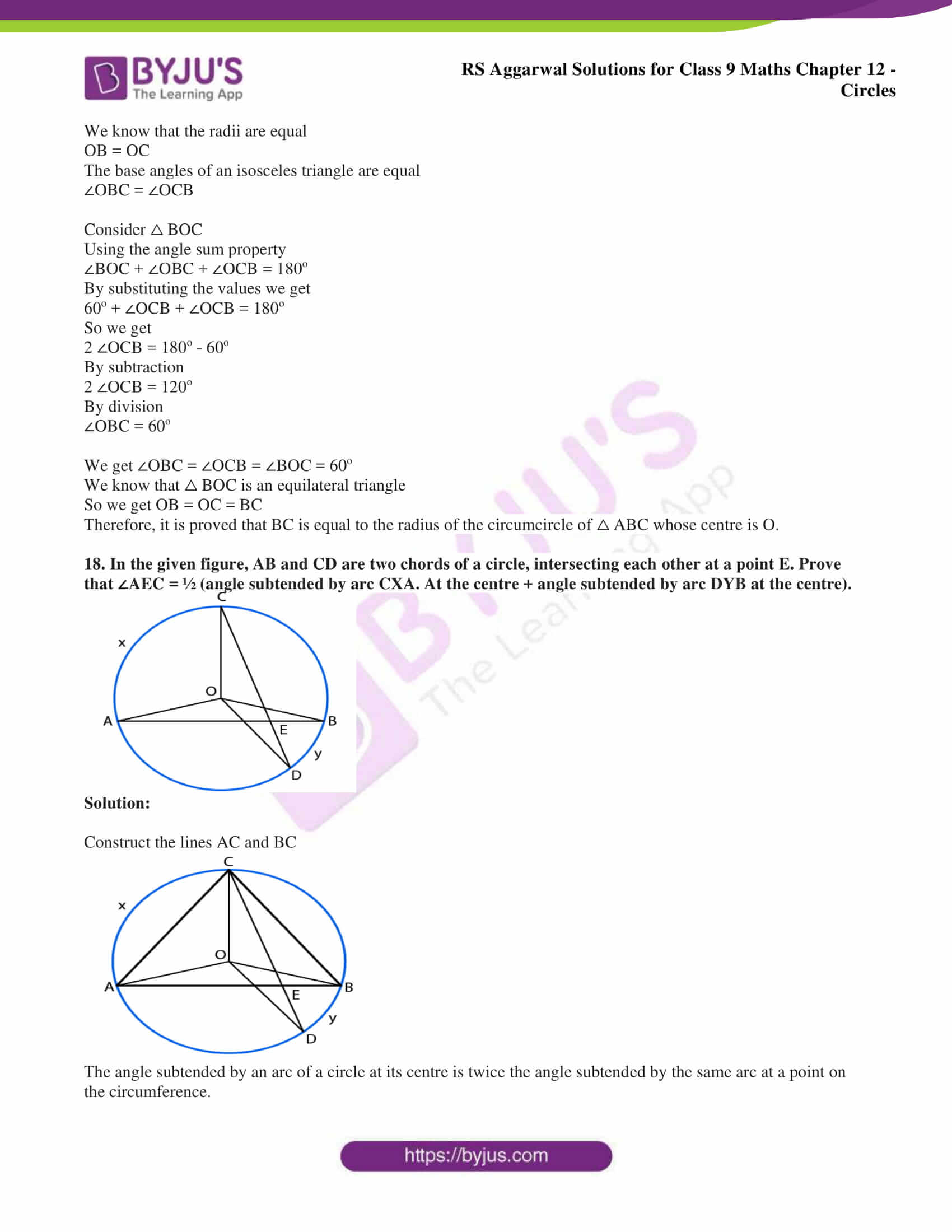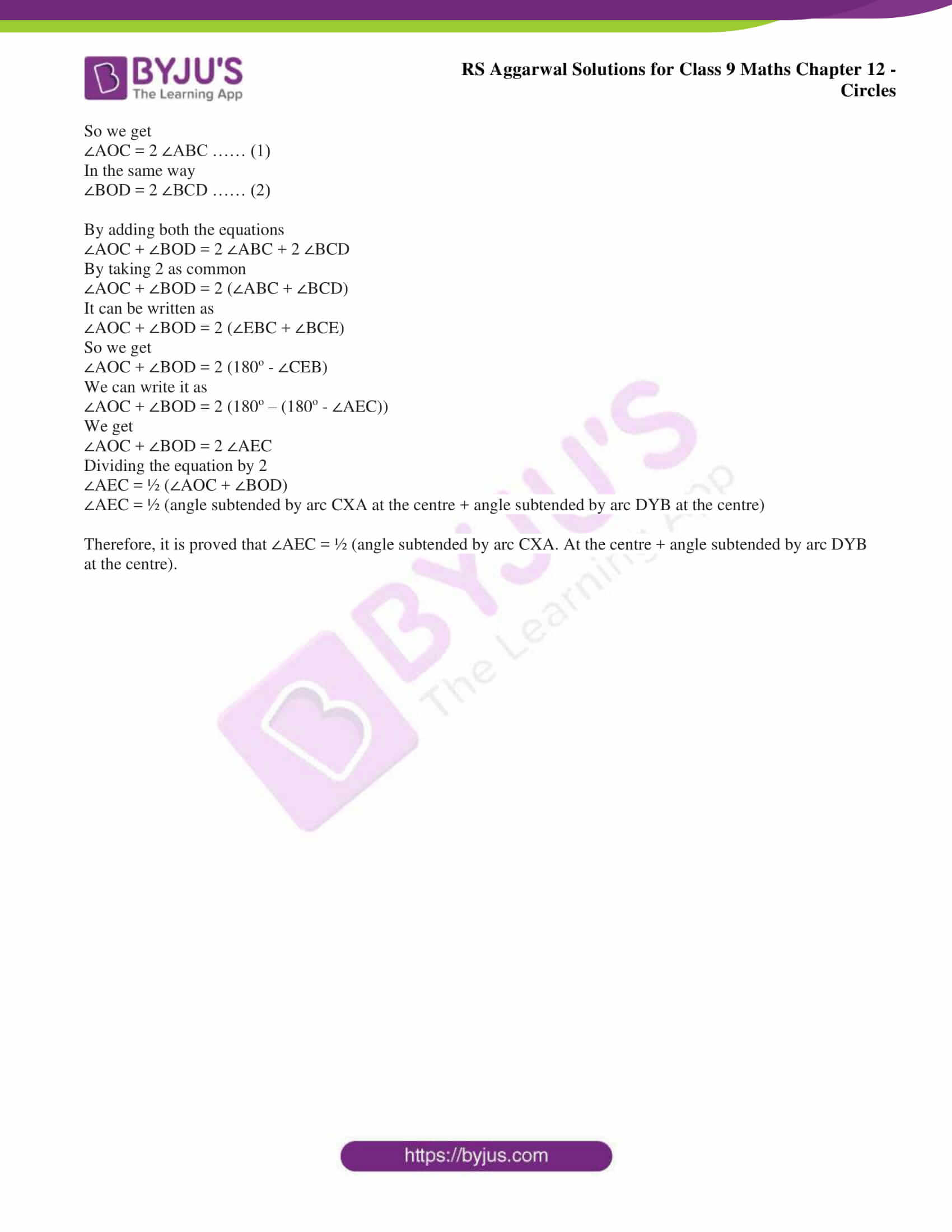## Access RS Aggarwal Solutions for Class 9 Chapter 12: Circles Exercise 12B

### Exercise 12(B) page: 456

1. (i) In Figure (1), O is the centre of the circle. If ∠OAB = 40o and ∠OCB = 30o, find ∠AOC.

(ii) In Figure (2), A, B and C are three points on the circle with centre O such that ∠AOB = 90o and

∠AOC = 110o. Find ∠BAC.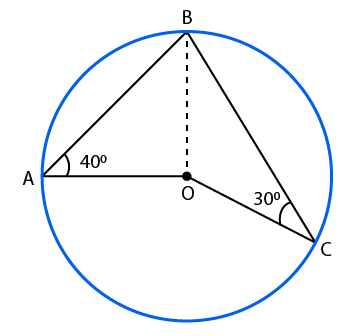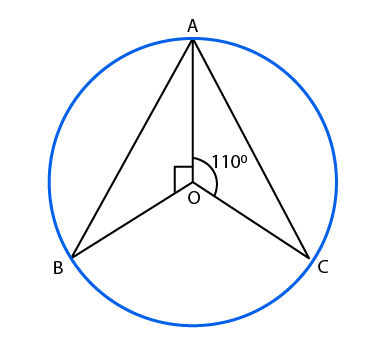Solution:

(i) Join the line BOConsider △ BOC

We know that the sides are equal to the radius

So we get

OC = OB

From the figure we know that the base angles of an isosceles triangle are equal

∠OBC = ∠OCB

It is given that

∠OCB = 30o

So we get

∠OBC = ∠OCB = 30o

So we get ∠OBC = 30o ……. (1)

Consider △ BOA

We know that the sides are equal to the radius

So we get

OB = OC

From the figure we know that the base angles of an isosceles triangle are equal

∠OAB = ∠OBA

It is given that

∠OAB = 40o

So we get

∠OAB = ∠OBA = 40o

So we get ∠OBA = 40o ……. (2)

We know that

∠ABC = ∠OBC + ∠OBA

By substituting the values

∠ABC = 30o + 40o

So we get

∠ABC = 70o

We know that the angle subtended by an arc of a circle at the centre is double the angle subtended by the arc at any point on the circumference.

It can be written as

∠AOC = 2 × ∠ABC

So we get

∠AOC = 2 × 70o

By multiplication

∠AOC = 140o

Therefore, ∠AOC = 140o

(ii) From the figure we know that∠AOB + ∠AOC + ∠BOC = 360o

By substituting the values

90o + 110o + ∠BOC = 360o

On further calculation

∠BOC = 360o – 90o – 110o

By subtraction

∠BOC = 360o – 200o

So we get

∠BOC = 160o

We know that

∠BOC = 2 × ∠BAC

It is given that ∠BOC = 160o

∠BAC = 160o/2

By division

∠BAC = 80o

Therefore, ∠BAC = 80o.

2. In the given figure, O is the centre of the circle and ∠AOB = 70o. Calculate the values of

(i) ∠OCA,

(ii) ∠OAC.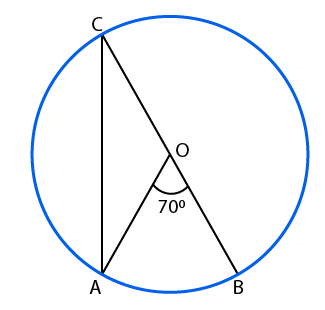Solution:

(i) We know that the angle subtended by an arc of a circle at the centre is double the angle subtended by the arc at any point on the circumference.

So we get

∠AOB = 2 ∠OCA

It is given that ∠AOB = 70o

We can write it as

∠OCA = 70o/2

By division

∠OCA = 35o

Therefore, ∠OCA = 35o.

(ii) From the figure we know that the radius is

OA = OC

We know that the base angles of an isosceles triangle are equal

∠OAC = ∠OCA

It is given that ∠OCA = 35o

So we get

∠OAC = 35o

Therefore, ∠OAC = 35o.

3. In the given figure, O is the centre of the circle. If ∠PBC = 25o and ∠APB = 110o, find the value of ∠ADB.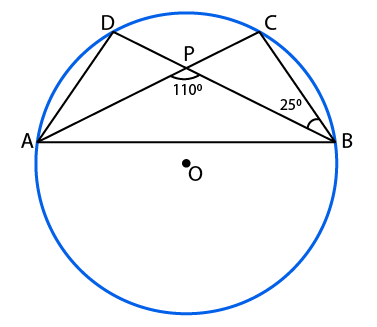Solution:

We know that ∠ACB = ∠PCBIn △ PCB

Using the angle sum property

∠PCB + ∠BPC + ∠PBC = 180o

We know that ∠APB and ∠BPC are linear pair

By substituting the values

∠PCB + (180o – 110o) + 25o = 180o

On further calculation

∠PCB + 70o + 25o = 180o

∠PCB + 95o = 180o

By subtraction

∠PCB = 180o – 95o

So we get

∠PCB = 85o

We know that the angles in the same segment of a circle are equal

Therefore, the value of ∠ADB is 85o.

4. In the given figure, O is the centre of the circle. If ∠ABD = 35o and ∠BAC = 70o, find ∠ACB.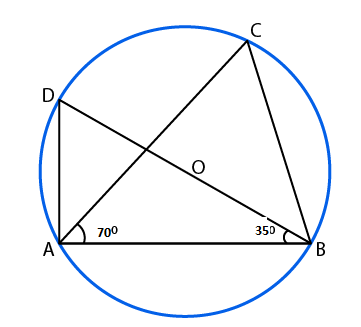Solution:

We know that BD is the diameter of the circle

Angle in a semicircle is a right angle

Using the angle sum property

By substituting the values

∠ADB + 90o + 35o = 180o

On further calculation

∠ADB = 180o – 90o – 35o

By subtraction

So we get

We know that the angles in the same segment of a circle are equal

So we get

∠ACB = 55o

Therefore, ∠ACB = 55o.

5. In the given figure, O is the centre of the circle. If ∠ACB = 50o, find ∠OAB.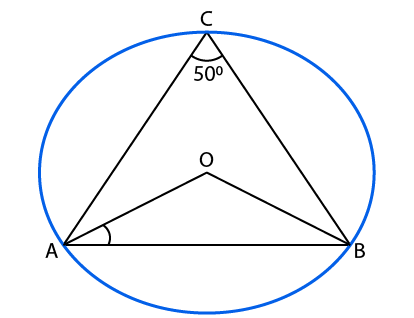Solution:

We know that the angle subtended by an arc of a circle at the centre is double the angle subtended by the arc at any point on the circumference.

So we get

∠AOB = 2 ∠ACB

It is given that ∠ACB = 50o

By substituting

∠AOB = 2 × 50o

By multiplication

∠AOB = 100o …… (1)

Consider △ OAB

We know that the radius of the circle are equal

OA = OB

Base angles of an isosceles triangle are equal

So we get

∠OAB = ∠OBA ……. (2)

Using the angle sum property

∠AOB + ∠OAB + ∠OBA = 180o

Using equations (1) and (2) we get

100o + 2 ∠OAB = 180o

By subtraction

2 ∠OAB = 180o – 100o

So we get

2 ∠OAB = 80o

By division

∠OAB = 40o

Therefore, ∠OAB = 40o.

6. In the given figure, ∠ABD = 54o and ∠BCD = 43o, calculate

(i) ∠ACD,

(iii) ∠BDA.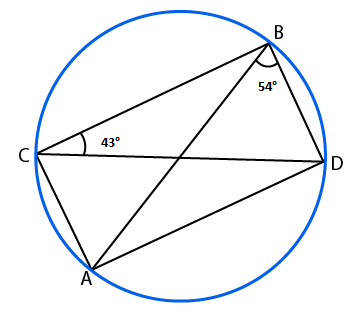Solution:

(i) We know that the angles in the same segment of a circle are equal

From the figure we know that ∠ABD and ∠ACD are in the segment AD

∠ABD = ∠ACD = 54o

(ii) We know that the angles in the same segment of a circle are equal

From the figure we know that ∠BAD and ∠BCD are in the segment BD

(iii) In △ ABD

Using the angle sum property

By substituting the values

43o + ∠ADB + 54o = 180o

On further calculation

∠ADB = 180o – 43o – 54o

By subtraction

So we get

It can be written as

∠BDA = 83o

7. In the adjoining figure, DE is a chord parallel to diameter AC of the circle with centre O. If ∠CBD = 60o, calculate ∠CDE.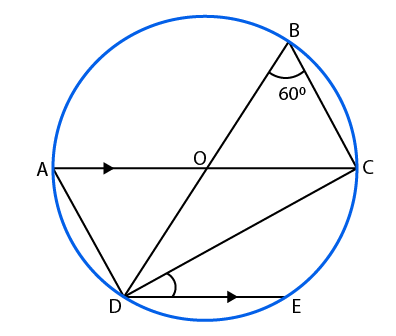Solution:

We know that the angles in the same segment of a circle are equal

From the figure we know that ∠CAD and ∠CBD are in the segment CD

An angle in a semi-circle is a right angle

So we get

Using the angle sum property

By substituting the values

∠ACD + 90o + 60o = 180o

On further calculation

∠ACD = 180o – 90o – 60o

By subtraction

∠ACD = 180o – 150o

So we get

∠ACD = 30o

We know that AC || DE and CD is a transversal

From the figure we know that ∠CDE and ∠ACD are alternate angles

So we get

∠CDE = ∠ACD = 30o

Therefore, ∠CDE = 30o.

8. In the adjoining figure, O is the centre of a circle. Chord CD is parallel to diameter AB. If ∠ABC = 25o, calculate ∠CED.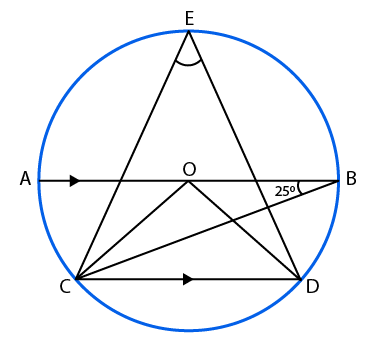Solution:

We know that ∠BCD and ∠ABC are alternate interior angles

∠BCD = ∠ABC = 25o

We know that the angle subtended by an arc of a circle at the centre is double the angle subtended by the arc at any point on the circumference.

∠BOD = 2 ∠BCD

It is given that ∠BCD = 25o

So we get

∠BOD = 2 (25o)

By multiplication

∠BOD = 50o

In the same way

∠AOC = 2 ∠ABC

So we get

∠AOC = 50o

From the figure we know that AB is a straight line passing through the centre

Using the angle sum property

∠AOC + ∠COD + ∠BOD = 180o

By substituting the values

50o + ∠COD + 50o = 180o

On further calculation

∠COD + 100o = 180o

By subtraction

∠COD = 180o – 100o

So we get

∠COD = 80o

We know that

∠CED = ½ ∠COD

So we get

∠CED = 80o/2

By division

∠CED = 40o

Therefore, ∠CED = 40o.

9. In the given figure, AB and CD are straight lines through the centre O of a circle. If ∠AOC = 80o and ∠CDE = 40o, find

(i) ∠DCE,

(ii) ∠ABC.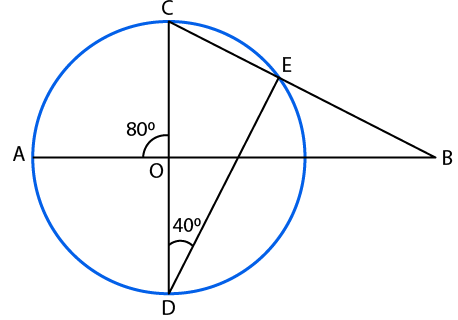Solution:

(i) From the figure we know that ∠CED = 90o

Consider △ CED

Using the angle sum property

∠CED + ∠EDC + ∠DCE = 180o

By substituting the values

90o + 40o + ∠DCE = 180o

On further calculation

∠DCE = 180o – 90o – 40o

By subtraction

∠DCE = 180o – 130o

So we get

∠DCE = 50o

(ii) We know that ∠AOC and ∠BOC form a linear pair

It can be written as

∠BOC = 180o – 80o

By subtraction

∠BOC = 100o

Using the angle sum property

∠ABC + ∠BOC + ∠DCE = 180o

By substituting the values

∠ABC + 100o + 50o = 180o

On further calculation

∠ABC = 180o – 100o – 50o

By subtraction

∠ABC = 180o – 150o

So we get

∠ABC = 30o

10. In the given figure, O is the centre of a circle, ∠AOB = 40o and ∠BDC = 100o, find ∠OBC.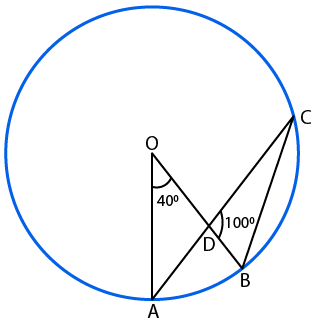Solution:

We know that the angle subtended by an arc of a circle at the centre is double the angle subtended by the arc at any point on the circumference.

So we get

∠AOB = 2 ∠ACB

From the figure we know that

∠ACB = ∠DCB

It can be written as

∠AOB = 2 ∠DCB

We also know that

∠DCB = ½ ∠AOB

By substituting the values

∠DCB = 40o/2

By division

∠DCB = 20o

In △ DBC

Using the angle sum property

∠BDC + ∠DCB + ∠DBC = 180o

By substituting the values we get

100o + 20o + ∠DBC = 180o

On further calculation

∠DBC = 180o – 100o – 20o

By subtraction

∠DBC = 180o – 120o

So we get

∠DBC = 60o

From the figure we know that

∠OBC = ∠DBC = 60o

So we get

∠OBC = 60o

Therefore, ∠OBC = 60o.

11. In the adjoining figure, chords AC and BD of a circle with centre O, intersect at right angles at E. If ∠OAB = 25o, calculate ∠EBC.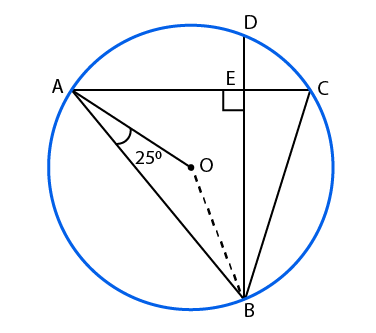Solution:

We know that OA and OB are the radius

Base angles of an isosceles triangle are equal

So we get

∠OBA = ∠OAB = 25o

Consider △ OAB

Using the angle sum property

∠OAB + ∠OBA + ∠AOB = 180o

By substituting the values

25o + 25o + ∠AOB = 180o

On further calculation

∠AOB = 180o – 25o – 25o

By subtraction

∠AOB = 180o – 50o

So we get

∠AOB = 130o

We know that the angle subtended by an arc of a circle at the centre is double the angle subtended by the arc at any point on the circumference

So we get

∠AOB = 2 ∠ACB

It can be written as

∠ACB = ½ ∠AOB

By substituting the values

∠ACB = 130/2

By division

∠ACB = 65o

So we get

∠ECB = 65o

In △ BEC

Using the angle sum property

∠EBC + ∠BEC + ∠ECB = 180o

By substituting the values

∠EBC + 90o + 65o = 180o

On further calculation

∠EBC = 180o – 90o – 65o

By subtraction

∠EBC = 180o – 155o

So we get

∠EBC = 25o

Therefore, ∠EBC = 25o.

12. In the given figure, O is the centre of a circle in which ∠OAB = 20o and ∠OCB = 55o. Find

(i) ∠BOC,

(ii) ∠AOC.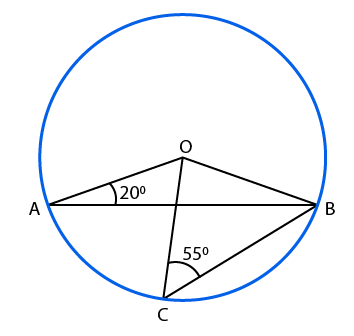Solution:

(i) We know that OB = OC which is the radius

The base angles of an isosceles triangle are equal

So we get

∠OBC = ∠OCB = 55o

In △ BOC

Using the angle sum property

∠BOC + ∠OCB + ∠OBC = 180o

By substituting the values

∠BOC + 55o + 55o = 180o

On further calculation

∠BOC = 180o – 55o – 55o

By subtraction

∠BOC = 180o – 110o

So we get

∠BOC = 70o

(ii) We know that OA = OB which is the radius

The base angles of an isosceles triangle are equal

So we get

∠OBA= ∠OAB = 20o

In △ AOB

Using the angle sum property

∠AOB + ∠OAB + ∠OBA = 180o

By substituting the values

∠AOB + 20o + 20o = 180o

On further calculation

∠AOB = 180o – 20o – 20o

By subtraction

∠AOB = 180o – 40o

So we get

∠AOB = 140o

We know that

∠AOC = ∠AOB – ∠BOC

By substituting the values

∠AOC = 140o – 70o

So we get

∠AOC = 70o

13. In the given figure, O is the centre of the circle and ∠BCO = 30o. Find x and y.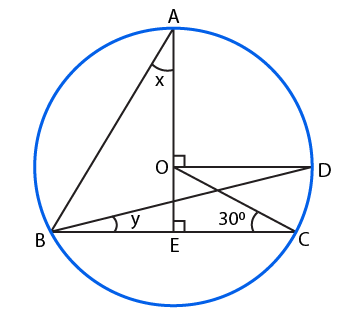Solution:

From the figure we know that ∠AOD and ∠OEC form right angles

So we get

∠AOD = ∠OEC = 90o

We know that OD || BC and OC is a transversal

From the figure we know that ∠AOD and ∠OEC are corresponding angles

∠AOD = ∠OEC

We know that ∠DOC and ∠OCE are alternate angles

∠DOC = ∠OCE = 30o

Angle subtended by an arc of a circle at its centre is twice the angle subtended by the same arc at a point on the circumference.

So we get

∠DOC = 2 ∠DBC

It can be written as

∠DBC = ½ ∠DOC

By substituting the values

∠DBC = 30/2

By division

y = ∠DBC = 15o

In the same way

∠ABD = ½ ∠AOD

By substituting the values

∠ABD = 90/2

By division

∠ABD = 45o

We know that

∠ABE = ∠ABC = ∠ABD + ∠DBC

So we get

∠ABE = ∠ABC = 45o + 15o

∠ABE = ∠ABC = 60o

Consider △ ABE

Using the angle sum property

∠BAE + ∠AEB + ∠ABE = 180o

By substituting the values

x + 90o + 60o = 180o

On further calculation

x = 180o – 90o – 60o

By subtraction

x = 180o – 150o

So we get

x = 30o

Therefore, the value of x is 30o and y is 15o.

14. In the given figure, O is the centre of the circle, BD = OD and CD ⊥ AB. Find ∠CAB.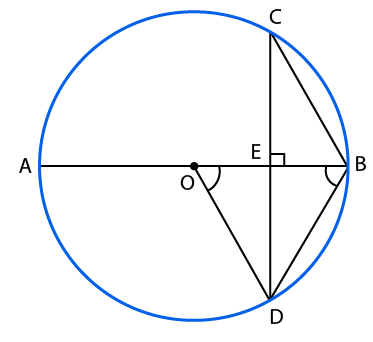Solution:

Join the points AC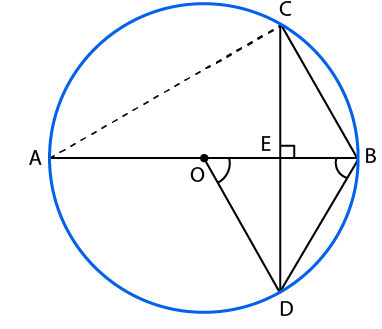It is given that BD = OD

We know that the radii of same circle are equal

OD = OB

It can be written as

BD = OD = OB

Consider △ ODB as equilateral triangle

We know that

∠ODB = 60o

The altitude of an equilateral triangle bisects the vertical angle

So we get

∠BDE = ∠ODE = ½ ∠ODB

By substituting the values

∠BDE = ∠ODE = 60/2

By division

∠BDE = ∠ODE = 30o

We know that the angles in the same segment are equal

∠CAB = ∠BDE = 30o

Therefore, ∠CAB = 30o.

15. In the given figure, PQ is a diameter of a circle with centre O. If ∠PQR = 65o, ∠SPR = 40o and ∠PQM = 50o, find ∠QPR, ∠QPM and ∠PRS.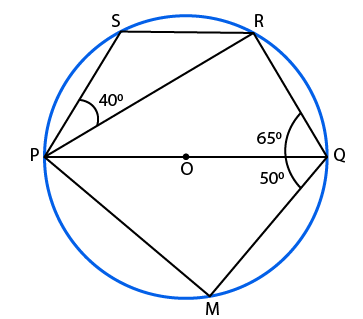Solution:

In △ PQR

We know that PQ is the diameter

So we get

∠PRQ = 90o as the angle in a semicircle is a right angle

Using the angle sum property

∠QPR + ∠PRQ + ∠PQR = 180o

By substituting the values

∠QPR + 90o + 65o = 180o

On further calculation

∠QPR = 180o – 90o – 65o

By subtraction

∠QPR = 180o – 155o

So we get

∠QPR = 25o

In △ PQM

We know that PQ is the diameter

So we get

∠PMQ = 90o as the angle in a semicircle is a right angle

Using the angle sum property

∠QPM + ∠PMQ + ∠PQM = 180o

By substituting the values

∠QPM + 90o + 50o = 180o

On further calculation

∠QPM = 180o – 90o – 50o

By subtraction

∠QPM = 180o – 140o

So we get

∠QPM = 40o

We know that

∠QPS + ∠SRQ = 180o

It can be written as

∠QPR + ∠RPS + ∠PRQ + ∠PRS = 180o

By substituting the values

25o + 40o + 90o + ∠PRS = 180o

On further calculation

∠PRS = 180o – 25o – 40o – 90o

By subtraction

∠PRS = 180o – 155o

So we get

∠PRS = 25o

16. In the figure given below, P and Q are centres of two circles, intersecting at B and C, and ACD is a straight line. If ∠APB = 150o and ∠BQD = xo, find the value of x.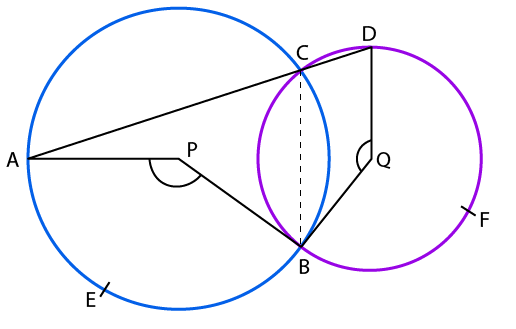Solution:

The angle subtended by an arc of a circle at its centre is twice the angle subtended by the same arc at a point on the circumference

So we get

∠APB = 2 ∠ACB

It can be written as

∠ACB = ½ ∠APB

By substituting the values

∠ACB = 150/2

So we get

∠ACB = 75o

We know that ACD is a straight line

It can be written as

∠ACB + ∠DCB = 180o

By substituting the values

75o + ∠DCB = 180o

On further calculation

∠DCB = 180o – 75o

By subtraction

∠DCB = 105o

We know that

∠DCB = ½ × reflex ∠BQD

By substituting the values

105o = ½ × (360o – x)

On further calculation

210o = 36o – x

By subtraction

x = 150o

Therefore, the value of x is 150o.

17. In the given figure, ∠BAC = 30o. Show that BC is equal to the radius of the circumcircle of △ ABC whose centre is O.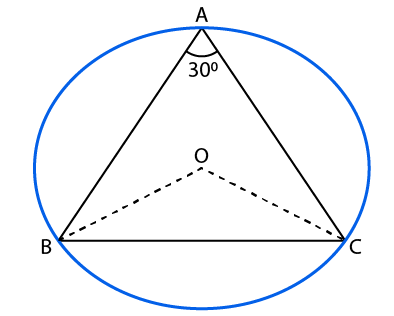Solution:

Join the lines OB and OC

We know that the angle subtended by an arc of a circle at the centre is double the angle subtended by the arc at any point on the circumference

So we get

∠BOC = 2 ∠BAC

It is given that ∠BAC = 30o

∠BOC = 2 × 30o

By multiplication

∠BOC = 60o

In △ BOC

We know that the radii are equal

OB = OC

The base angles of an isosceles triangle are equal

∠OBC = ∠OCB

Consider △ BOC

Using the angle sum property

∠BOC + ∠OBC + ∠OCB = 180o

By substituting the values we get

60o + ∠OCB + ∠OCB = 180o

So we get

2 ∠OCB = 180o – 60o

By subtraction

2 ∠OCB = 120o

By division

∠OBC = 60o

We get ∠OBC = ∠OCB = ∠BOC = 60o

We know that △ BOC is an equilateral triangle

So we get OB = OC = BC

Therefore, it is proved that BC is equal to the radius of the circumcircle of △ ABC whose centre is O.

18. In the given figure, AB and CD are two chords of a circle, intersecting each other at a point E. Prove that ∠AEC = ½ (angle subtended by arc CXA. At the centre + angle subtended by arc DYB at the centre).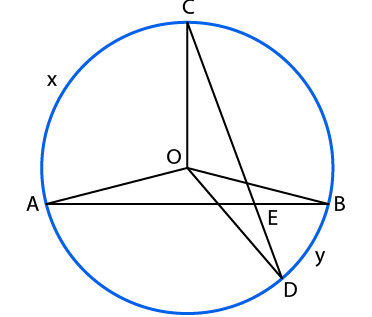Solution:

Construct the lines AC and BC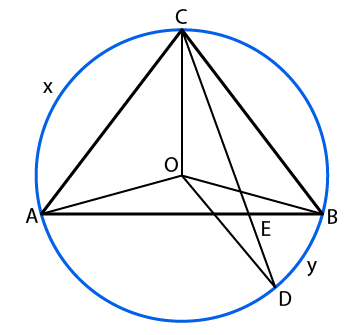The angle subtended by an arc of a circle at its centre is twice the angle subtended by the same arc at a point on the circumference.

So we get

∠AOC = 2 ∠ABC …… (1)

In the same way

∠BOD = 2 ∠BCD …… (2)

∠AOC + ∠BOD = 2 ∠ABC + 2 ∠BCD

By taking 2 as common

∠AOC + ∠BOD = 2 (∠ABC + ∠BCD)

It can be written as

∠AOC + ∠BOD = 2 (∠EBC + ∠BCE)

So we get

∠AOC + ∠BOD = 2 (180o – ∠CEB)

We can write it as

∠AOC + ∠BOD = 2 (180o – (180o – ∠AEC))

We get

∠AOC + ∠BOD = 2 ∠AEC

Dividing the equation by 2

∠AEC = ½ (∠AOC + ∠BOD)

∠AEC = ½ (angle subtended by arc CXA at the centre + angle subtended by arc DYB at the centre)

Therefore, it is proved that ∠AEC = ½ (angle subtended by arc CXA. At the centre + angle subtended by arc DYB at the centre).

### Access other exercise solutions of Class 9 Maths Chapter 12: Circles

Exercise 12A Solutions 23 Questions

Exercise 12C Solutions 26 Questions

### RS Aggarwal Solutions Class 9 Maths Chapter 12 – Circles Exercise 12B

RS Aggarwal Solutions Class 9 Maths Chapter 12 Circles Exercise 12B contains the theorems which are based on the results of angles which are subtended by arcs and problems regarding them.

### Key features of RS Aggarwal Solutions for Class 9 Maths Chapter 12: Circles Exercise 12B

• Studying concepts in Mathematics is made simple with the help of RS Aggarwal Solutions in PDF format.
• The solutions are in a stepwise manner which improves problem solving abilities among students.
• Several examples before each exercise mainly help students gain conceptual knowledge before solving the problems.
• Theorems are explained in brief as each of them carry more marks in the board exam according to the CBSE syllabus.